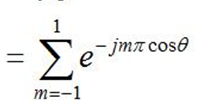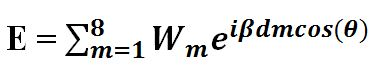# help in exp function in matlab

Status
Not open for further replies.

#### Mohammed EL_Kenawy

##### Newbie level 5
Hey

Can you help me plz to plot the output of this equation using Matlabthe vertical axes is ...... Magnitude of the output in dB
the Horizontal axes is the angle in degrees

could I have the code of this

I really need it

help me

Last edited:This is a real function.

Only three terms: m= -1,0,1 ==> Σ(...) = 1 + 2*cos(pi*cos(θ))

thank you Eduardo but how about theta .
I want it in degrees 0 20 40 60 .... 180 on xlabel but i have an error
I can't fix the dimensions of length
can u help me ?

thank you Eduardo but how about theta .
I want it in degrees 0 20 40 60 .... 180 on xlabel but i have an error

Something like this ?

Code:
t = 0:.5:180 ;                  % 0.5° step
plot(t,20*log10(abs(y)))        % 20*log10(abs(...  convert to decibels
xlabel('Degrees')               % axis labels
ylabel('dB')

•Mohammed EL_Kenawy

### Mohammed EL_Kenawy

Points: 2
Thank you very much Eduardo

for this equation i want to plot E(indB) versus theta (in degrees)say W =[ 0 0.2857 0.5714 0.8571 0.8571 0.5714 0.2857 0]

I want the code to see if it give the figure

plz I need the code my friend

I have an error with undefined function w
I used for loop but can't run
help me

It's the same, just take care with the matrix multiplication.

Code:
beta = 1 ; d = 1 ;   % Dummy values

W =[ 0 0.2857 0.5714 0.8571 0.8571 0.5714 0.2857 0] ;
m = 1:8 ;
angle = 0:.5:180 ;   % 0.5° step
z = exp(i*beta*d*m'*cos(angle*pi/180)) ;

plot(angle,20*log10(abs(W*z)))
xlabel('Degrees')
ylabel('dB')

•Mohammed EL_Kenawy

### Mohammed EL_Kenawy

Points: 2
@Mohammed EL_Kenawy: Perhaps you could post your matlab code so that someone can give some hints.

Thank you very much Eduardo